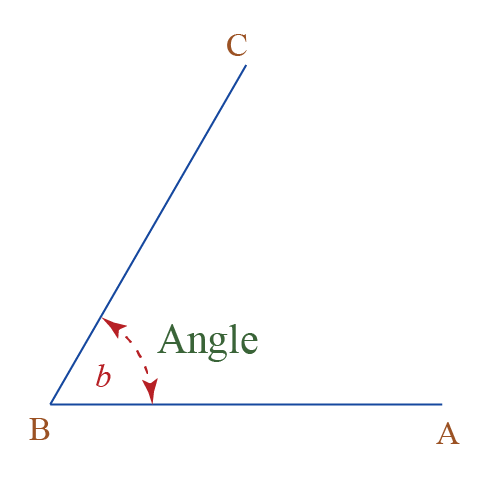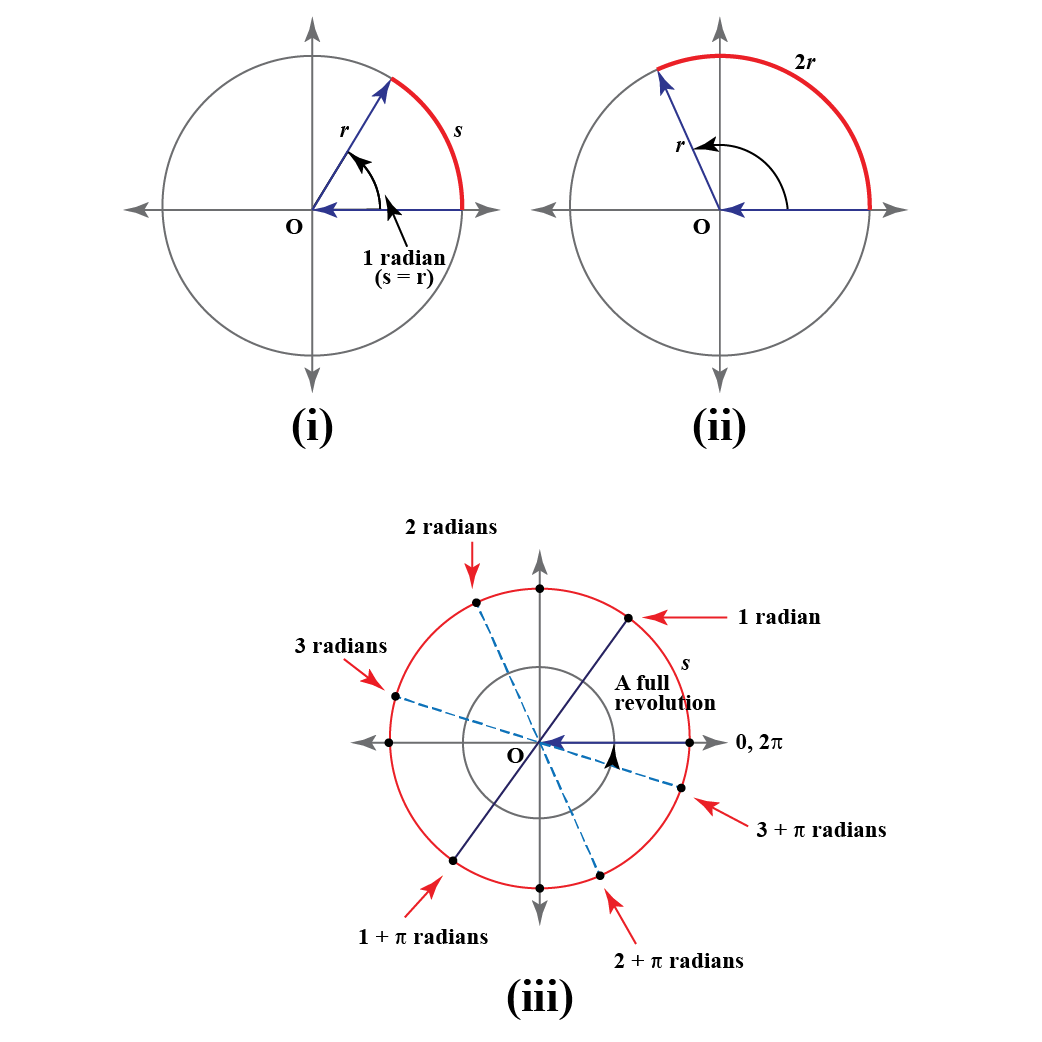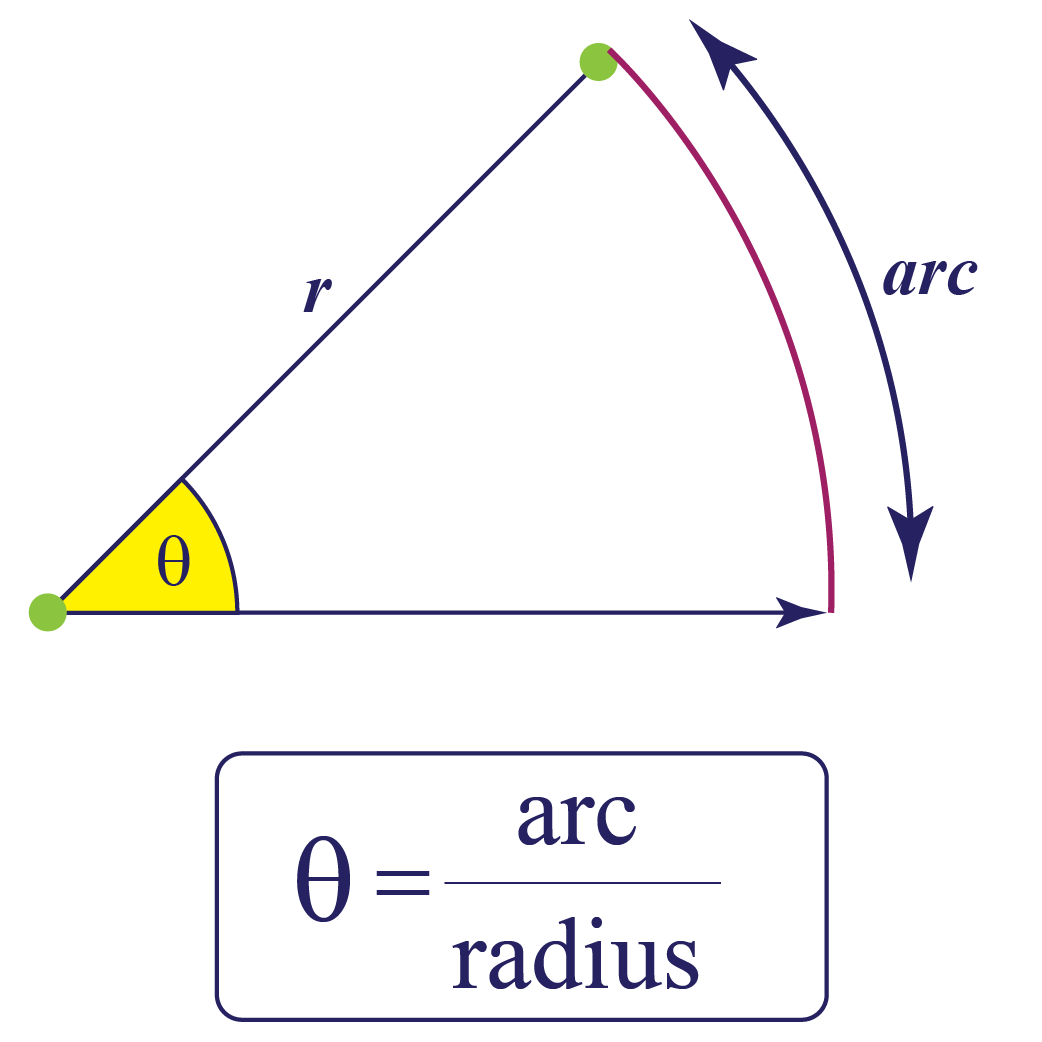This chapter will cover the various concepts on the subject including the radian formula, the radian definition, the radian calculator, and radian vs degrees.

Up to this stage, you have been using degrees to measure the sizes of angles.However, for a variety of reasons, angle measures in advanced mathematics are frequently described using a unit system different from the degree-system. This system is known as the radian system.

Did you know that radian was the SI supplementary unit for measuring angles before 1995? It was later changed to a derived unit.

## Lesson Plan

 1 What Is a Radian? 2 Tips and Tricks 3 Solved Examples on Radian 4 Challenging Questions on Radian 5 Interactive Questions on Radian

Radian is a unit to measure angles.

We use radians in place of degrees when we want to calculate the angle in terms of radius.

As '$$^{\circ}$$' is used to represent degree, rad or $$^c$$ is used to represent radians. For example, 1.5 radians is written as 1.5 rad or 1.5c.

### Definition

1 radian is an angle subtended by the arc of length r(radius of the same circle) at the center.

Consider this situation:

Take the radius of the circle, wrap it around the circle.

Now the angle subtended at the center is 1 radian.

###The angle subtended by the half-circle is $$180^{\circ}$$ which is equal to $$\pi \text { radian}$$.

And the angle subtended by one complete counterclockwise revolution is equal to $$2 \pi \text { radian}$$.

The radian is also defined as the ratio of the arc length to the radius of the circle:$$\theta = \dfrac { \text {arc}}{ \text { radius}}$$

Here, both the lengths are measured in the same unit.

## Why Do We Use Radian?

The most important reason why we use radian because it is a dimensionless measure.

Dimensionless quantities are usually defined as a ratio of two similar quantities. Since it is a ratio of two terms hence the unit of two similar quantities gets canceled and is therefore equal to 1. However, unit 1 is not written with dimensionless quantities.

A dimensionless measure such as radian is convenient to use as it makes calculations easier.

For example, if we want to find the derivative of trigonometric functions, it is simple to use radians rather than degrees.

Using radians, the derivative of sine function is-

$\dfrac d {dx} \sin x = \cos x$

And if the derivative is calculated in degrees, it comes as-

$\dfrac d {dx} \sin x = \dfrac {\pi}{180} \cos x$

Additional thing in the result makes it very hard to solve.

Hence we use radian just to save our time and remove the extra terms in the calculation.

## What Is the Difference Between Radian and Degrees?

Radians and degrees both measure angles where 1 radian is equal to 57.2958 degrees.

Let us see how 1 radian is equal to 57.2958 degrees-

Since the angle made by the half-circle is 180 degrees, it also equals to $$\pi$$ radians.

Hence, \begin{align*} \pi \text { radian} &= 180 \text { degrees} \\ 1 \text { radian} &= \dfrac {180}{ \pi} \text { degrees } \\ &= 57.2958 \text { degrees } \end{align*}

The equivalent degree and radian values can be seen in the following table:

30$$^{\circ}$$ $$\dfrac { \pi}{6}$$
45$$^{\circ}$$ $$\dfrac { \pi}{4}$$
60$$^{\circ}$$ $$\dfrac { \pi}{3}$$
90$$^{\circ}$$ $$\dfrac { \pi}{2}$$
180$$^{\circ}$$ $$\pi$$
270$$^{\circ}$$ $$\dfrac { 3 \pi}{2}$$
360$$^{\circ}$$ $$2 \pi$$

## Usage

• Angles are most or generally measured in radians in calculus and in most other branches of mathematics.
• Radians are widely used in physics also. They are preferred over degrees when angular measurements are done in physics.Tips and Tricks

• You can convert the degree into the radian;
Just multiply by $$\dfrac {\pi} {180}$$.
• You can also convert the radian into the degree;
Just multiply by $$\dfrac {180}{\pi}$$.

## Solved Examples

 Example 1

Convert the following angles into radians.

1. $$120^{\circ}$$
2. $$150^{\circ}$$

Solution

1. $120^{\circ} = \dfrac {\pi} {180} \times 120 = \dfrac 23 \pi$
2. $150^{\circ} = \dfrac {\pi} {180} \times 150 = \dfrac 56 \pi$
 $$\therefore$$ $$120^{\circ} = \dfrac 23 \pi$$ $$150^{\circ} = \dfrac 56 \pi$$
 Example 2

Sean wants to know the length of the arc whose radius is 5 in and the angle subtended by the arc is 1.5 radian. Can you help her with this?

Solution

Given, r = 5 in and $$\theta$$ = 1.5rad.

\begin{align*} \theta &= \dfrac { \text {arc}}{ \text { radius}} \\ or \ \, \text {arc} &= \theta \times \text {radius } \end{align*}

$$\implies$$$\text {arc} = 1.5 \times 5 = 7.5 \text{ in}$

 $$\therefore$$ Length of the arc = 7.5 in
 Example 3

Ema was given a task to convert the $$\dfrac 79 \pi$$ radian into degrees. Can you tell her the right process to convert it?

Solution

We can convert the radian into the degree, by just multiplying by $$\dfrac {180}{\pi}$$.

$\dfrac 79 \pi = \dfrac {180}{\pi} \times \dfrac 79 \pi = 140^{\circ}$

 $$\therefore \dfrac 79 \pi = 140^{\circ}$$
 Example 4

A pendulum of length 18 in oscillates at an angle of 38 degrees. Find the length of the arc that it covers.

Solution

Given, length of the pendulum(r) = 18 in

$\theta = 38^{\circ} = 38 \times \dfrac {\pi}{180}$

Since, \begin{align*} \text {length of the arc } &= \theta \times r \\ \implies \, \, \, \, \, \, &= 38 \times \dfrac {\pi}{180} \times 18 \\ &= 3.8\pi\end{align*}

 $$\therefore$$ Length of the arc = $$3.8 \pi$$.
 Example 5

A wire of length 60 in is bent to form an arc of radius 40 in. Find the angle subtended at the center by this arc.

Solution

Given, the length of the arc = 60 in, and the radius of the arc = 40 in.

\begin{align*} \theta &= \dfrac { \text {arc}}{ \text { radius}} \\ \theta &= \dfrac { 60}{40} \\ &= \dfrac 32 \text{rad} \end{align*}

 $$\therefore \theta = \dfrac 32 \text{ rad}$$Challenging Questions
• How would you experimentally calculate the value of π using a given circle?

## Interactive Questions

Here are a few activities for you to practice. Select/Type your answer and click the "Check Answer" button to see the result.

## Let's Summarize

This mini-lesson targeted the fascinating concept of radians. The math journey around radian starts with what a student already knows, and goes on to creatively crafting a fresh concept in the young minds. Done in a way that not only it is relatable and easy to grasp, but also will stay with them forever. Here lies the magic with Cuemath.

At Cuemath, our team of math experts is dedicated to making learning fun for our favorite readers, the students!

Through an interactive and engaging learning-teaching-learning approach, the teachers explore all angles of a topic.

Be it worksheets, online classes, doubt sessions, or any other form of relation, it’s the logical thinking and smart learning approach that we, at Cuemath, believe in.

## 1. What is the radians to degree formula?

To convert degrees into radians, multiply the degrees by $$\pi 180^{\circ}$$. For converting radians into degrees just multiply by $$\dfrac {180}{\pi}$$.

## 2. How do you simplify radian?

You can convert the radian into the degrees, just multiply by $$\dfrac {180}{\pi}$$.

Now simplifying the equation further simplifies the problem.

## 3. What is the measure of 0 in radian?

0 degrees is equal to 0 radians.

• Angles are most or generally measured in radians in calculus and in most other branches of mathematics.
• Radians are widely used in physics also. They are preferred over degrees when angular measurements are done in physics.
More Important Topics
Numbers
Algebra
Geometry
Measurement
Money
Data
Trigonometry
Calculus